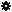﻿ Object Model: PolygonMesh.GetTriangleVertexIndexArray

PolygonMesh.GetTriangleVertexIndexArray

## PolygonMesh.GetTriangleVertexIndexArrayv5.0

### Description

Returns the vertex indices of the polygon subtriangle on which the point locators are defined.

Each vertex index triplet is guaranteed to correspond to one polygon (the one returned by PolygonMesh.GetPolygonIndexArray). The barycentric position within the triangle can be queried with PolygonMesh.GetTriangleWeightArray.

Warning: This information may in some cases vary between geometry instances having the same topology but different deformations. Since the point locators are defining a precise position on the surface, and since deformations can dynamically change the triangulation within a polygon, it can happen that a point locator is remapped to a different subtriangle (both barycentric weights and subtriangle indices can vary).

An example using this method can be found in PolygonMesh.GetPolygonIndexArray and Geometry.GetClosestLocations methods' description.

### C# Syntax

 ```Object PolygonMesh.GetTriangleVertexIndexArray( PointLocatorData in_pPtLocators, Object in_pPointLocatorsIndices ); ```

### Scripting Syntax

 ```oArray = PolygonMesh.GetTriangleVertexIndexArray( PointLocators, [Indices] ); ```

### Return Value

2-dimensional Array of index triplets

### Parameters

Parameter Type Description
PointLocators PointLocatorData Point locators to be described.
Indices 1-dimensional Array Indices of the point locators to be described (all in not specified).